# Introduction To Value At Risk

Large institutions deal with immense amounts of currencies which enter and leave their accounts on a daily bases. Furthermore, they have their own funds that it has to efficiently allocate so as to maximize their return on investment but also wish to hedge against adverse events. With certain confidence, the entities (particularly commercial institutions) should be conscious of their profits and also know how much they stand to lose.  With requirements stipulating certain amounts to be kept for times of need, the introduction of capital buffers which should build up in prosperous times (SARB, 2015), as well as other regulatory matters institutions, have increased the need to know how much they possess and the risks they are exposed to.

In recent years the trading accounts at large commercial banks have grown substantially and become progressively more diverse and complex (Jorion, 2001). To manage market risks, major trading institutions have developed large scale risk measurement models. While approaches may differ, all such models measure and aggregate market risks in current positions at a highly detailed level.

Value-at-Risk (VaR) provides a comprehensive solution to these and many more concerns. Value-at-risk model measures market risk by determining how much the value of a portfolio could decline over a given period of time with a given probability as a result of changes in the market prices or rates. (Hendricks, 1996). In portfolio allocation terms; VaR is simply a standard deviation calculation, which illustrates how volatile a portfolio is (Butler, 1999).

## Determining VaR

When it comes to the VaR number; one should be cautious in using it for inference making as it is determined primarily by how the designer of the risk-management system wants to interpret it (Linsmeier & Pearson, 2000). Three such approaches obtaining VaR are Historical Simulation, the Delta Normal approach and the Monte Carlo Simulation.

### Historical Simulation

In essence, the approach involves using historical changes in market rates and prices to construct a distribution of potential future portfolio profits and losses and then reading off the VaR  (Linsmeier & Pearson, 2000, p. 50)from this distribution. One can use the historical method by mining a database of actual historical returns unadjusted as the source for simulated returns and extracting inference figures from them, this will capture the correlations, volatilities tail, fatness and skewness in returns that are actually present in the data avoiding a need to parameterize and estimate a mathematical model (Pan & Duffie, 1997).

An advantage of the historical simulation is inherent inclusion of all events i.e. crashes and other rare events as such the effects of these events are incorporated in the calculation since it makes use of actual historical returns.

### Delta-Normal Approach

The delta-normal approach is based on the assumption that the underlying market factors have a multivariate normal distribution. One can determine the distribution of mark-to-market portfolio profits and losses, which is also assumed to be normal. Once the distribution of possible portfolio profits and losses has been obtained, standard mathematical properties of the normal distribution are used to determine the loss that will be equalled or exceeded x per cent of the time i.e. VaR.

### Monte Carlo Simulations

The Monte Carlo simulation methodology has a number of similarities to historical simulation. Monte Carlo simulation allows the risk manager to use actual historical distributions for risk factor returns rather than having to assume normal returns. A large number of randomly generated simulations are run forward in time using volatility and correlation estimates chosen by the risk manager (Linsmeier & Pearson, 2000). Various algorithms and methods have been developed for this purpose but most require extensive computation and programming skills.

## Testing the VaR Model

There is no one VaR number for a single portfolio because different methodologies used for calculating VaR produce different results. Which then would be the best method of obtaining VaR? If the observations are independent and identical distributed then, tests like the Kullback discrepancy or Kolmogorov-Smirnov tests may be used to test the validity of the model. Since managers stipulate which factors have a greater impact on their portfolios, the best choice will be determined by which dimensions the risk manager considers most important.

Hendricks further goes on to mention that “to most important components of VaR models are the length of time over which the market risk is to be measured and the confidence level at which the market risk is measured” (1996,p. 40). By accounting for adjusting these components a manager will be able to attain enhanced results.

## Computing VaR

Bo in his book on Value at Risk proposed four methods computing the VaR number: using the distribution of the portfolio return; the rate of portfolio return; delta-normal valuation and the delta-gamma method (2001, p. 4). For illustration, I will only discuss the first two methods and leave the latter for the reader to explore.

### Distribution of the portfolio return

Obtaining the VaR value is as simple as solving the equation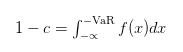Where c is our confidence level and ƒ(x) is a density function that reflects the change in portfolio value.ƒ(x) can be any function as such for our analysis we will assume is normally distributed with mean μ and variance σ².

Example:

We want to know how much we stand to lose if we buy stock Χ. moreover, we want to be 95% certain of this value. Assume Χ trades with μ = 0.3  and σ² = 0.4.

From the normal tables, 95% certainty is obtained for a value Χ  in which Χ`s z-score is equal to 1.645. The transformation equation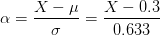Therefore we have Χ = σα + μ = 1.645 * 0.633 + 0.3 = 1.341

Next, we look at VaR in a portfolio context.

### Rate of portfolio return

Suppose the current portfolio value is Ρand produces a rate of return ℜ. Let the Ρ0 be normally distributed with mean μ and standard deviation σ. After one period the portfolio value will be given by Ρ1 = Ρ0(1 + ℜ) with mean Ρ0(1 + μand variance Ρ0σ. Let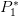be the lowest value the portfolio can reach with a certain level of confidence

The value at risk is then calculated as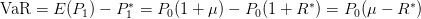If for some reason we choose mean equal to 0 then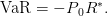Jorion proposed the substitution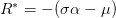(2001, pp.110-113) which we can plug in our VaR calculation to give the following: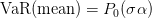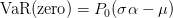## Where and How VaR is applied

Returning on our bank scenario; we have that all the requirements mentioned are stipulated it what is known as the Basel accords. The Basel II Accord was designed to monitor and encourage sensible risk-taking using appropriate models of risk to calculate Value-at-Risk (VaR)  (Chang, Jimenez-Martins, McAleer, & Perez-Amaral, 2001). Regulatory bodies like the South African Reserve Bank make use of VaR figures to assess how stable an institution is and evaluates whether the institution’s risk is appropriately managed.

Besides being used in large institutional levels, VaR calculations are applied in portfolio constructions and management.  Greater volatility is induced by greater risk in some underlying variables or by the design of products that are more sensitive to financial products. This is particularly true when dealing with options as their worth is dependent on some underlying price. A manager may use VaR to asses her risk exposure and calibrate it accordingly to meet her investment needs.

## Conclusion

VaR has established itself as a key building block of financial risk management (Jorion, 2001). To implement VaR, all of a firm’s positions data must be gathered into one centralized database. Once this is complete the overall risk has to be calculated by aggregating the risks from individual instruments across the entire portfolio. But determining which method to use to obtain this Value-at-Risk is a different objective in itself as the one to compute must be aware of the variables that will produce a meaningful VaR figure.

Three approaches which may be used to calculate VaR are the Delta normal approach, Monte Carlo and Historic simulations. The approaches above can be solely used to get the VaR figure but normally the manager may use all three to get a better understanding of his business dynamics and there is that reminder that different compliance requirements oblige the manager to report different VaR figures.

## Bibliography

• Butler, C. (1999). Mastering Value at Risk: A step-by-step guide to understanding and applying VaR.Trowbridge, Wiltshire: Pitman Publishing.
• Chang, C.-l., Jimenez-Martins, J.-A., McAleer, M., & Perez-Amaral, T. (2001). Risk Management of Risk under the Basel Accord: Forecasting Value-at-Risk.
• Hendricks, D. (1996). Evaluation of Value-at-Risk Using Historical Data.
• Jorion, P. (2001). Value At Risk: The new benchmark for managing Financial risk. New York: McGraw Hill.
• Linsmeier, T. J., & Pearson, N. D. (2000). Value at Risk. Financial Analysts Journal, 47-67.
• Pan, J., & Duffie, D. (1997). An Overview of Value at Risk.
• SARB. (n.d.). South Africa’s implementation of Basel II and Basel III. Retrieved June 23, 2015, from SARB: https://www.resbank.co.za
• YieldCurve.com. (2003). An Introduction to Value-at-Risk.

Disclaimer: The views, opinions, and information provided within this guest post are those of the author alone and do not represent those of QuantInsti®. The accuracy, completeness, and validity of any statements made or the links shared within this article are not guaranteed. We accept no liability for any errors, omissions or representations. Any liability with regards to infringement of intellectual property rights remains with them.